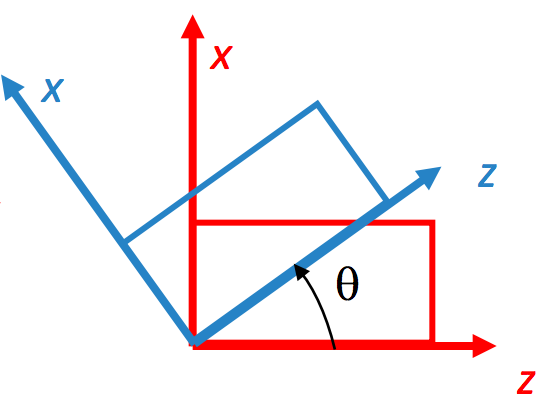Kinematics - Rigid Body Transformation

Core Math in Robotics Kinematics following course MEAM 620 at Penn

January 29, 2017 - 3 minute read -

Rigid Body Transformations

Notations

• $R_{C}^A$ represents the orientation of frame $C$ with respect to frame $A$.
• $T_{C}^A$ represents the translation of frame $C$ with respect to frame $A$.
• $H_{C}^A$ represents the homogeneous transformations of frame $C$ with respect to frame $A$.

Where, in 3d, $R_{C}^A$ is a 3 $\times$ 3 matrix, $T_{C}^A$ is a $3\times 1$ vector.

• $R_{x,\phi},R_{y,\theta}, R_{z, \psi}$ represent rotations about the axis (right-hand rule) by angleComposite Transformations

• Post-multiply successive transformations about intermediate frames
• Pre-multiply successive transformations about fixed frames

Composition of homogeneous transformations follows the rules of rotation matrices.

Inverse Transformations

• Rotations
• Homogeneous transformations

Skew Symmetric Matrices

$% $ is the skew symmetrix matrix.

For any skew symmetrix matrix $A^T = -A$.

2D Rotation

The rotation in 2d can be viewed as the complex number $Z(\theta) = cos(\theta) +i sin(\theta)$

Rodrigues’ Formula

Rodrigues’ formula gives us an decomposition of the rotation matrix into axis and angle.

Quaternions

Quatornion is a 4 dimensional representation of the rotation matrix. The basis are $\{1, i, j, k\}$. The quatornion is a generalization of complex number.

• Unit Quatornion Properties
• Relation to angle-axis

• Not unique

• Commutativity

• Conjugate

• Inverse

• Norm

• Multiplication

• Operation on a vector ($q$ is representing rotation matrix $R$)